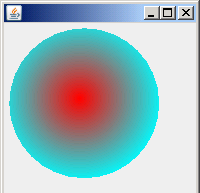Tutorials

The radial gradient defines a color at a point, and blends into another color. We are providing you an example which displays the radial gradient.

The radial gradient defines a color at a point, and blends into another color. We are providing you an example which displays the radial gradient.

The radial gradient defines a color at a point, and blends into another color. We are providing you an example which displays the radial gradient. The RoundGradientPaint  class describes the center of the gradient, radius and background color with the defined points and colors. The RoundGradientPaint class returns a RoundGradient from its createContext() method. The gradient blends color from the center point to the background color.

To translate a pixel value into color components and an alpha component, class ColorModel is used. The class AffineTransform provides transparency, scaling, rotation, shearing.

The getTransparency() method returns the translucent or opaque depending on the color specified that were passed to the constructor of the class RoundGradientPaint. The dispose() method is called when the PageContext is no longer needed.

To calculate the distance from the center point and iterates over each point in the ellipse, the getRaster() method is used. The class WritableRaster provide pixel writing capabilities.

Here is the code of RoundGradientPaintExample.java

 ``` import java.awt.*; import javax.swing.*; import java.awt.geom.*; import java.awt.image.*; public class RoundGradientPaintExample extends JPanel{  public void paint(Graphics g) {   Graphics2D g2d = (Graphics2D) g;   Ellipse2D ellipse = new Ellipse2D.Float(5, 5, 150, 150);   RoundGradientPaint roundGradientPaint = new RoundGradientPaint(    75, 75, Color.red,new Point2D.Double(0, 85), Color.cyan);   g2d.setPaint(roundGradientPaint);   g2d.fill(ellipse);   }  public static void main(String[] args) {   JFrame frame = new JFrame();   frame.getContentPane().add(new RoundGradientPaintExample());   frame.setSize(200, 200);   frame.show();   }  class RoundGradientPaint implements Paint {  Point2D point1;  Point2D point2;  Color color1, color2;  public RoundGradientPaint(double x, double y, Color pcolor,   Point2D radius, Color bcolor) {   point1 = new Point2D.Double(x, y);    color1 = pcolor;   point2 = radius;   color2 = bcolor;   }  public PaintContext createContext(ColorModel colorModel,     AffineTransform affineTransform) {   Point2D transPoint = affineTransform.transform(point1, null);   Point2D transRadius = affineTransform.deltaTransform(point2, null);   return new RoundGradient(transPoint, color1,transRadius, color2);   }   public int getTransparency() {   int a = color1.getAlpha();   int b = color2.getAlpha();   return (((a & b) == 0xff) ? OPAQUE : TRANSLUCENT);   }   }   public class RoundGradient implements PaintContext {   Point2D point3, point4;   Color color3, color4;   public RoundGradient(Point2D p, Color c1, Point2D r, Color c2) {   point3 = p;   color3 = c1;   point4 = r;   color4 = c2;   }  public void dispose() {}  public ColorModel getColorModel() {   return ColorModel.getRGBdefault();   }  public Raster getRaster(int p, int q, int width, int height) {   WritableRaster writableRaster = getColorModel()    .createCompatibleWritableRaster(width, height);   int[] data = new int[width * height * 4];   for (int j = 0; j < height; j++) {   for (int i = 0; i < width; i++) {   double distance = point3.distance(p + i,q + j);   double radius = point4.distance(0, 0);   double num = distance / radius;   if (num > 1.0)   num = 1.0;   int value = (j * width + i) * 4;   data[value + 0] = (int) (color3.getRed() + num   * (color4.getRed() - color3.getRed()));   data[value + 1] = (int) (color3.getGreen() + num   * (color4.getGreen() - color3.getGreen()));   data[value + 2] = (int) (color3.getBlue() + num   * (color4.getBlue() - color3.getBlue()));   data[value + 3] = (int) (color3.getAlpha() + num   * (color4.getAlpha() - color3.getAlpha()));   }   }   writableRaster.setPixels(0, 0, width, height, data);   return writableRaster;   }   } }```

Output will be displayed as: